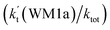## Catalytic effect of (H2O)n (n = 1–3) on the HO2 + NH2 → NH3 + 3O2 reaction under tropospheric conditions†

### Abstract

The effects of (H2O)n (n = 1–3) clusters on the HO2 + NH2 → NH3 + 3O2 reaction have been investigated by employing high-level quantum chemical calculations with M06-2X and CCSD(T) theoretical methods, and canonical variational transition (CVT) state theory with small curvature tunneling (SCT) correction. The calculated results show that two kinds of reaction, HO2⋯(H2O)n (n = 1–3) + NH2 and H2N⋯(H2O)n (n = 1–3) + HO2, are involved in the (H2O)n (n = 1–3) catalyzed HO2 + NH2 → NH3 + 3O2 reaction. Due to the fact that HO2⋯(H2O)n (n = 1–3) complexes have much larger stabilization energies and much higher concentrations than the corresponding complexes of H2N⋯(H2O)n (n = 1–3), the atmospheric relevance of the former reaction is more obvious with its effective rate constant of about 1–11 orders of magnitude faster than the corresponding latter reaction at 298 K. Meanwhile, due to the effective rate constant of the H2O⋯HO2 + NH2 reaction being respectively larger by 5–6 and 6–7 orders of magnitude than the corresponding reactions of HO2⋯(H2O)2 + NH2 and HO2⋯(H2O)3 + NH2, the catalytic effect of (H2O)n (n = 1–3) is mainly taken from the contribution of the water monomer. In addition, the enhancement factorof the water monomer is 10.06–13.30% within the temperature range of 275–320 K, which shows that at whole calculated temperatures, a positive water effect is obvious under atmospheric conditions.## Article information

Article type
Paper
Submitted
03 Aug 2018
Accepted
23 Oct 2018
First published
05 Nov 2018### Permissions### Catalytic effect of (H2O)n (n = 1–3) on the HO2 + NH2 → NH3 + 3O2 reaction under tropospheric conditions

T. Zhang, K. Wang, Z. Qiao, Y. Zhang, L. Geng, R. Wang, Z. Wang, C. Zhao and L. Jin, RSC Adv., 2018, 8, 37105 DOI: 10.1039/C8RA06549G